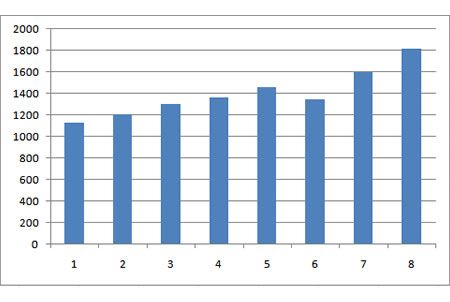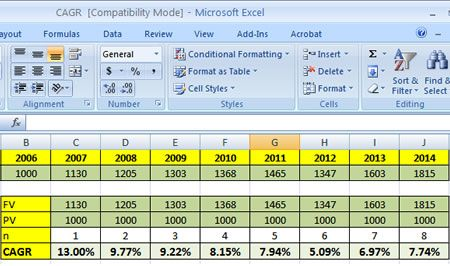# Calculate Annualised Return Using Compound Annual Growth Rate (CAGR)

Compound Annual Growth Rate measures the yearly compounded rate of investment growth over a period of time. It computes the smoothed rate of investment return for a specified number of years.Simply put, if an investment generated a 10% annual compounded return over a 5-year period, it means that the investment had grown 10% per year over each of the 5 years at an even pace to arrive at the final value although the actual growth rate may not equivalent to 10% each year over that 5-year period.

Let’s take an example:

– Revenue grow from RM1000 in one year to RM1500 in the next. How much growth is that?

– And what if sales grow from RM1000 to RM1500 over 3 years. How much growth is that?

Example of Simple Growth Calculation

To calculate simple growth, subtract the final value from the base value and divide the result by the base value. Then, multiply by 100 if you want to show it in %.

(1500-1000) / 1000 = 500 / 1000 = 0.5

((1500-1000) / 1000) * 100 = 50%

Example of Annual Compound Growth Rate (CAGR) CalculationThe growth rate is about 9% per year over 3 years when the value has grown from RM1000 in the first year to RM1303 in the third.

The formula to calculate CAGR:

(final value / base value) ^ (1/periods)-1

The CAGR is often used to measure a company’s growth against its historical performance or that of other companies from the same time periods. It gives a clear picture of the company’s performance over the longer term as it is not only takes into account the effects of compounding but also smoothen the ups and downs of both the market cycles from year to year.